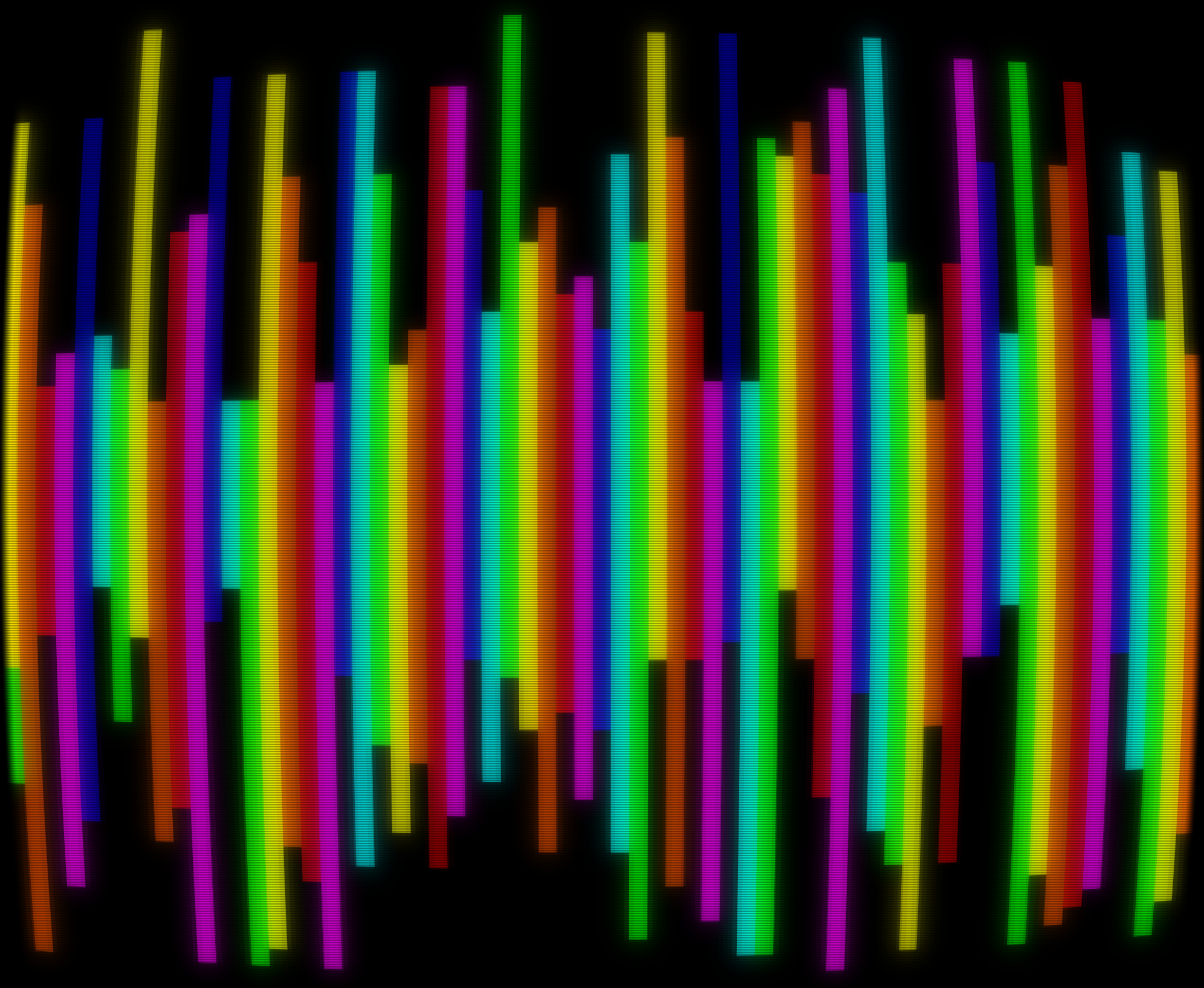## / schazers / games / Bit Waveform

Jason Riggs @schazers  ·  1 year ago
require 'bits' N,j,z=65,0,.05 function _D() for i=0,N do V(i,17+RN(-11,11),49+RN(-11,11),(i+j)%7+5) end end function _U(dt) if T(1)>z then j=j-1 RT(1) end end